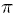1. Fundamental theorem

Find the derivative.Find the derivative.Let.
Evaluate F().

need some direction on these bad johnnys...thanks

mathlete

2. $\displaystyle g(x) = \int\limits_{h(x)}^{j(x)} {f(t)dt} + C\quad \Rightarrow \quad g'(x) = f\left( {j(x)} \right)j'(x) - f\left( {h(x)} \right)h'(x)$

Search Tags

fundamental, theorem